## Atomic Structure

Early in the study of atomic structure, Sir Joseph Thomson and Ernest Rutherford produced competing models of the atom. Thomson proposed the “plum‐pudding” model. In this model, the negative charges— electrons (the plums)—were surrounded by the positive charges (the pudding), filling the volume of the atom.

To demonstrate his model, Rutherford bombarded thin metal foils with a beam of positively charged particles. Most of the particles went through with little effect, but occasionally one was deflected through a large angle. By way of explanation, Rutherford proposed a planetary model of the atom with the negatively charged electrons orbiting a central concentration of positive charge—the nucleus.

Two main difficulties occur in the planetary model of the atom. First, any object moving in a circle is accelerating. According to classical mechanics, an accelerating charge radiates energy, producing electromagnetic waves; therefore, the orbiting electron should radiate energy and fall quickly into the nucleus. Because matter does exist, this obviously does not happen. Second, an atom emits only certain electromagnetic radiation, not a continuum of all frequencies.

## Atomic spectra

When a gas is excited by a spark, light of a particular color is produced. For example, neon gas produces a red‐orange color. When this light is spread through a prism, a series of bright lines of specific wave lengths are observed, called a line spectrum. The line spectra are characteristics of a certain gas, rather like its atomic fingerprint. For example, the visible line spectrum of hydrogen consists primarily of wavelengths of approximately 656 nm, 486 nm, 434 nm, and 410 nm. Although it was not understood at the time why it worked, the following equation was found to describe the series of lines, called the Balmer series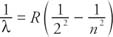where n is an integer of 3, 4, 5, … and R is a constant, now called the Rydberg constant: R = 1.0973732 × 10 7 m −1.

In addition to emitting light of certain wavelengths, an element can also absorb light of specific wavelengths. The light from a light bulb or the sun forms a continuous spectrum—the colors of the rainbow. When a continuous spectrum light is passed through a cool gas, the gas absorbs the same wavelengths that it emits when excited, which appear as a set of black lines in the continuous spectrum. The black lines are called the absorption spectrum.

## The Bohr atom

Niels Bohr (1852–1962) combined classical mechanics and some revolutionary postulates to formulate a model of the hydrogen atom that hoped to circumvent some of the difficulties of classical physics and still explain atomic spectra. The following are his basic postulates.

• Postulate 1: The electron moves in only certain permitted circular orbits—quantized states—around the positive nucleus under the influence of the Coulomb force.
• Postulate 2: The electron does not emit energy when it is in one of the allowed orbits called a stationary state.
• Postulate 3: When the electron jumps from one permitted state to another, the energy is given off as a particular photon with energy equal to the difference in the energies of the initial and final states:Planck's concepts of quantization can be seen in Bohr's postulates 1 and 3. When the electron is in a stationary state, Bohr assumed that Newton's laws, Coulomb's law, and conservation of energy were valid. Bohr showed that the angular momentum of an electron with mass m traveling with speed v about a circular orbit of radius r is quantized aswhere n is an integer and h is Planck's constant.

Also, he derived an expression for the radius of hydrogen from the electrostatic force (Coulomb's law) set equal to the centripetal force: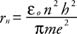When n = 1, the radius is called the Bohr radius, which is the smallest orbit of hydrogen.

To find an expression for the total energy of the electron orbiting the atom, use the classical formula for total energy, then substitute r n from above and v from the angular momentum to get the following: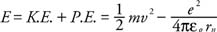When n = 1, the lowest energy state of the atom is called the ground state. The value of the ground state of hydrogen is −13.6 electron volts, which is in excellent agreement with the experimentally observed hydrogen ionization energy—the energy necessary to remove an electron in the ground state from an atom.

Combining this result with the equation in Bohr's postulate 2 yields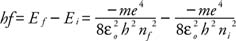Because c = f λ, the equation becomes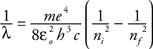From the preceding equation, the Rydberg constant may be calculated.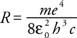All of these constants are known, and the theoretical value for the Rydberg constant is the same as this derived R. This demonstrated agreement is remarkable, and it validated Bohr's postulates.

## Energy levels

The Balmer series, found experimentally, can be explained by the Bohr model of the atom in the following way. Figure 1 is a diagram of the energy transitions possible for hydrogen.Figure 1 Energy transitions of a hydrogen atom, with the spectral series.

For the Balmer series, the hydrogen electron jumps from an initial excited state ( n = 3,4,5, …) to a final state at the n = 2 level. In so doing, it emits a photon with energy equal to the energy difference of the initial and final states. Other series indicated on Figure illustrate the other series of lines found by Theodore Lyman and Louis Paschen. This type of diagram is called an energy level diagram because it illustrates the discrete, allowed energy levels and the permissible transitions for the orbiting electron.

## De Broglie waves and the hydrogen atom

The next task was to suggest why only certain discrete energy levels are possible. De Broglie assumed that an orbit would be stable only if it contained a whole number or multiples of a whole number of electron de Broglie waves. Figure shows a representation of a standing circular wave of three wave lengths.Figure 2 Energy transitions of a hydrogen atom, with the spectral series.

The depicted orbit would be the permissible orbit with the quantum number of 3, that is, n = 3. This visual way of understanding quantization shows that the wave nature of matter is basic to a model of the atom. More complicated formulations of quantum physics that were developed later have built on these concepts.